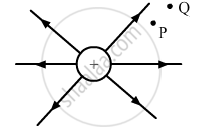Share

# The Figure Shows the Field Lines on a Positive Charge. is the Work Done by the Field in Moving a Small Positive Charge from Q to P Positive Or Negative? Give Reason. - Physics

ConceptElectric Field Lines

#### Question

The figure shows the field lines on a positive charge. Is the work done by the field in moving a small positive charge from Q to P positive or negative? Give reason.#### Solution

Work done = q.(potential at Q − potential at P), where q is the small positive charge
The electric potential at a point distant r due to the field created by a positive charge Q is given by

$V = \frac{1}{4 \pi\epsilon_0}\frac{q}{r}$

$\because r_P < r_Q$

$\Rightarrow V_P > V_Q$

∴ Work done will be negative.
Is there an error in this question or solution?

#### Video TutorialsVIEW ALL 

Solution The Figure Shows the Field Lines on a Positive Charge. is the Work Done by the Field in Moving a Small Positive Charge from Q to P Positive Or Negative? Give Reason. Concept: Electric Field Lines.
S